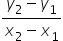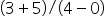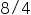Mathematics
Easy

Question

# Write the equation of the line that passes through the points (0, -5) and (4, 3).

## y=2x-5y=1/2x-5y=2x+5y=1/2x+5Hint:

## The correct answer is: y=2x-5

### Given points are (0, -5) and (4, 3).Step1 :  slope of the line is (m) =Slope of line === 2Step 2 :     Let the y-intercept be bThe slope-intercept form of equation of the line is y=mx+bSince the line passes through (0, -5), so, the y-intercept of the line is -5b= -5So, the equation of the line is y=2x-5

if x = 0 , then the corresponding value of y will be intercept.

### Related Questions to study#### With Turito Foundation.#### Get an Expert Advice From Turito.